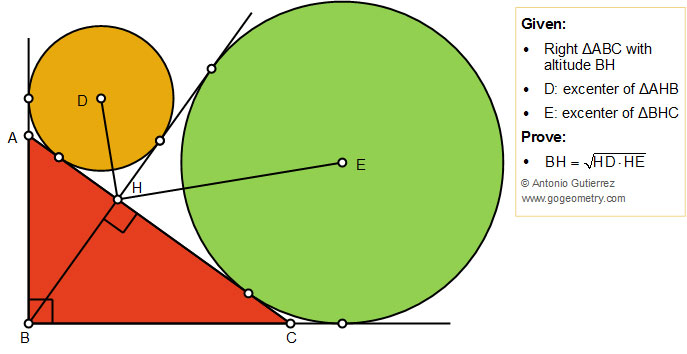Geometry Problem 1436: Right Triangle, Altitude, Excircles, Excenters, Geometric Mean, Art, PosterProposition

The figure shows a right triangle ABC with the altitude BH. D and E are the excenters of triangles AHB and BHC, respectively. Prove that BH is the geometric mean of HD and HE that is: $$BH = \sqrt{HD.HE}$$.